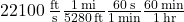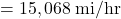## A satellite orbits earth at a speed of 22100 feet per second (ft/s). Use the following facts to convert this speed to miles per hour (mph).

Question

A satellite orbits earth at a speed of 22100 feet per second (ft/s). Use the following facts to convert this speed to miles per hour (mph). 1 mile = 5280 ft 1 min = 60 sec 1 hour = 60 min

in progress 0
7 months 2021-07-17T12:19:16+00:00 1 Answers 1 views 0